## Multiple Linear Regression

Problem statement: Find a relation between multiple independent variables and a dependent variable

Variables:

Independent Variables : Age, BMI, Children, Region, Expenses

Dependent Variable : smoker

# Importing the libraries

import numpy as np

import matplotlib.pyplot as plt

import pandas as pd

# Importing the dataset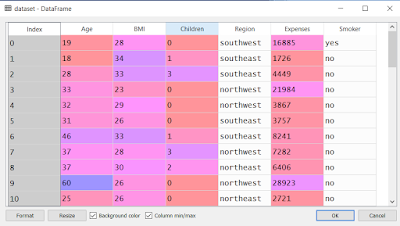# Separating Independent and Dependent variables

X = dataset.iloc[:, :-1].values

y = dataset.iloc[:, 5].values

# Encoding categorical data

import sklearn

from sklearn.preprocessing import LabelEncoder, OneHotEncoder

labelencoder_X = LabelEncoder()

X[:, 3] = labelencoder_X.fit_transform(X[:, 3])

onehotencoder = OneHotEncoder(categorical_features = )

X = onehotencoder.fit_transform(X).toarray()

labelencoder_y = LabelEncoder()

y = labelencoder_y.fit_transform
(y)

We have encoded the data which had 4 different values i.e. southwest, southeast, northwest, northeast under the variable "Region" and hence we've got 4 different columns.

We have also encoded the dependent variable i.e. "Smoker".# Avoiding the Dummy Variable Trap

X = X[:, 1:]

To avoid dummy trap, we need to remove one of the categorical variable. So, we removed the first column (encoded categorical variable).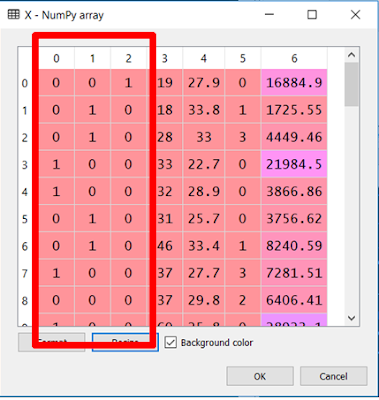# Splitting the dataset into the Training set and Test set

from sklearn.cross_validation import train_test_split

X_train, X_test, y_train, y_test = train_test_split(X, y, test_size = 0.2 , random_state = 0)# Fitting Multiple Linear Regression to the Training set

from sklearn.linear_model import LinearRegression

regressor = LinearRegression()

regressor.fit(X_train, y_train)

# Predicting the Test set results

y_pred = regressor.predict(X_test)

By comparing y_pred and y_test, we get to know that most of the values match and that our model is pretty good.# Building the optimal model using Backward Elimination

Multiple Linear Regression Equation : y = B0 + B1x1 + B2x2 +.....+ Bnxn

Here, B0 is the constant & x1, x2, xn are the independent variables.

Notice that B0 is not associated with any independent variable. So, we associate it with x0 = 1.

To do that, we have to add a column of 50 rows ( as our table has 50 data values) with all values=1.

X = np.append(arr = np.ones((50,1)).astype(int), values = X, axis = 1)

Now, we will try to remove some variables which do not have a great impact in predicting the dependent variable.

Step 1 : Set a significance value (eg. 0.05)

Step 2 : Check out the values of P for all the variables.

Step 3 : Find the max value of P and the corresponding X value (i.e. column index)

Step 4 : If P > 0.05, Remove that particular column and run the model again.

Step 5 : Keep repeating Steps 2 to 5 till you get all the P values < 0.05

For this, we will make X_opt and include all the variables in it. After running the model, we will remove the variable (which has the highest P value) from the array and run the model again.

import statsmodels.formula.api as sm

X_opt = X[:, [0, 1, 2, 3, 4, 5, 6, 7]]

regressor_OLS = sm.OLS(endog = y, exog = X_opt).fit()

regressor_OLS.summary()Here, the highest P value is 0.746 which is corresponding to x3. So, we need to remove the 3rd variable from the array. Start the count from 0 to 3 (0 because of constant). 3rd variable comes out to be 3. Hence, we remove 3 from the array and again run the model.

X_opt = X[:, [0, 1, 2, 4, 5, 6, 7]]

regressor_OLS = sm.OLS(endog = y, exog = X_opt).fit()

regressor_OLS.summary()Here, 0.680 is the highest P value which is corresponding to x5. We need to remove the 5th variable from the above array. Start the count from 0 to 5 in the above array i.e. X[:, [0, 1, 2, 4, 5, 6, 7]].

The 5th variable comes out to be 6. So, remove 6 and run the model again.

X_opt = X[:, [0, 1, 2, 4, 5, 7]]

regressor_OLS = sm.OLS(endog = y, exog = X_opt).fit()

regressor_OLS.summary()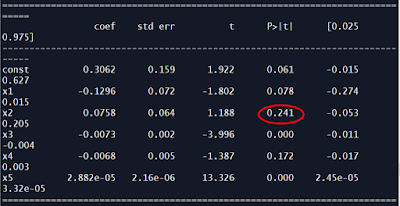Here, 0.241 is the highest P value which is corresponding to x2. So, we need to remove the 2nd variable from the above array. Start the count from 0 to 2 in the above array i.e. X[:, [0, 1, 2, 4, 5, 7]]

The 2nd variable comes out to be 2. So, remove 2 and run the model again.

X_opt = X[:, [0, 1, 4, 5, 7]]

regressor_OLS = sm.OLS(endog = y, exog = X_opt).fit()

regressor_OLS.summary()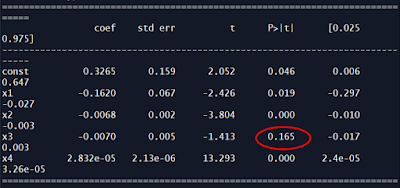Here, 0.165 is the highest P value which is corresponding to x3. We need to remove the 3rd variable from the above array. Start the count from 0 to 3 in the above array i.e. X[:, [0, 1, 4, 5, 7]].

The 3rd variable comes out to be 5. So, remove 5 and run the model again.

X_opt = X[:, [0, 1, 4, 7]]

regressor_OLS = sm.OLS(endog = y, exog = X_opt).fit()

regressor_OLS.summary()Here, 0.084 is the highest P value which is corresponding to constant i.e. x0. We need to remove the 0th variable from the above array. Start the count from 0 in the above array i.e. X[:, [0, 1, 4, 7]].

The 0th variable comes out to be 0. So, remove 0 and run the model again.

X_opt = X[:, [1, 4, 7]]

regressor_OLS = sm.OLS(endog = y, exog = X_opt).fit()

regressor_OLS.summary()Here, 0.067 is the highest P value which is corresponding to x1. We need to remove the 1st variable from the above array. Start the count from 1 (as there is no constant now) in the above array i.e. X[:, [1, 4, 7]].

The 1st variable comes out to be 1. So, remove 1 and run the model again.

X_opt = X[:, [4, 7]]

regressor_OLS = sm.OLS(endog = y, exog = X_opt).fit()

regressor_OLS.summary()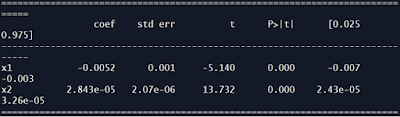Now, all the P values are less than the significance level i.e. 0.05.